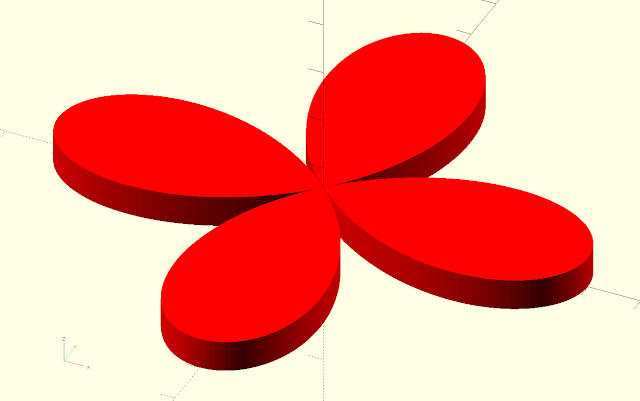## Introduction

In my previous blog post I explained how to create complex 2D shapes in OpenSCAD. Once a mathematical expression for a shape is known it's possible to translate it to OpenSCAD script. The Carthesian coordinates of mathematical rose, a rose shaped sinusoid, can be expressed by x = cos(kθ)cos(θ) and y = cos(kθ)sin(θ). If k is an integer a the shape will be relatively simple but is k is a fracture more complex shapes are created.One of the shapes that can be created with the rose_points function (linear_extrude is used to create a 3D shape).

## Writing the script

All the points needed to create the rose will be generated in the function rose_points. Rose
_points returns these points in a list. The function will have the form:

The parameters k and n will determine the shape of the rose. If n = 1 the curve will a simple rose shape but if n > 1 more complex shapes are drawn. Here is a link to all the possible shapes when varying k and n. The syntax of OpenSCAD for lists allows us to use if and for elements to construct a list. In the case of our function we can use these elements.

step = 1;
function rose_points(k, n , radius) = k%2 == 0 && n%2 ==1 ? [for (theta = [0 : step : 360 * n]) [radius * cos(k/n*theta) *sin(theta), radius * cos(k/n*theta) * cos(theta)]] : [for (theta = [0 : step : 180 * n]) [radius * cos(k/n*theta) * sin(theta), radius * cos(k/n*theta) * cos(theta)]];

a=rose_points(5,7,100);
color("red") polygon(a);

The function looks intimidating at first due to the list comprehensions.  The key is to just break it apart. The two parts between square brackets contain lists of points (generated by a for loop). The rest is an if, then, else statement: if k is even and n is uneven then [] else []. More information on the syntax for a list (list comprehensions) can be found here.

The function isn't perfect. With some combination of k and n (e.g. 6 and 2) nothing is displayed on the screen. The reason is that with this combination all points are repeated prompting the polygon function of OpenSCAD to draw nothing. In this cases it helps to change the range of theta.

Caveat: List comprehensions as shown in the rose_points function are only possible with OpenSCAD v2015.03 and above.

OpenSCAD is open source (GPLv2 license) and is well maintained by Marius Kintel et al. Besides the stable releases for Windows, OSX and Linux, development snapshots are available. I recommend using these development snapshots since they have all the latest features.Shape created with k = 2 and n = 1.Shape created with k =8 and n = 7.